# ISEE Lower Level Math : How to find the decimal equivalent of a fraction

## Example Questions

1 2 3 4 5 6 7 9 Next →

### Example Question #81 : Fractions

Find the decimal equivalent to, rounding to the nearest hundredth.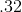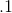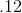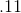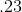Explanation:

To find the decimal equivalent of a fraction, divide the numerator by the denominator: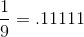repeating

Round to:### Example Question #82 : Fractions

What is the decimal of?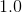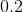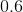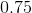Explanation:

The first step to turn a fraction to a decimal is to change the denominator to.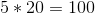so you must the same operation to the numerator to get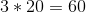.

Then take a decimal and place it in front of the numerator and your answer is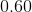.

### Example Question #83 : How To Find The Decimal Equivalent Of A Fraction

Write as a decimal: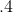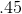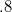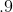Explanation:

To turn a fraction into a decimal, divide the numerator by the denominator.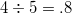### Example Question #483 : Numbers And Operations

What is the decimal equivalent of?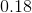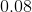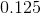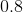Explanation:

To change a fraction to a decimal, you must make the denominatorand do the same operation to the numerator.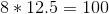(You can find that value by dividingby the denominator) so you must multiple the numerator by the same giving us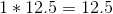.

You move the decimal in the numerator over two spaces to the left to get your decimal of.

### Example Question #81 : How To Find The Decimal Equivalent Of A Fraction

Writeas a fraction.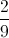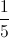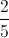Explanation:

First we rewrite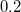as a fraction. Since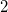is in the tenths place, we can write it over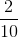Reduce the fraction:1 2 3 4 5 6 7 9 Next →

### All ISEE Lower Level Math Resources